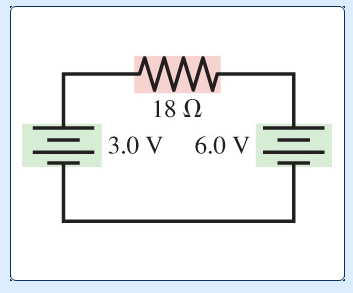# Problem: a. What is the magnitude of the current in the 18 Ω resistor in the figure? b. What is the direction of the current—to the left or to the right?

###### FREE Expert Solution

a. Voltage, V = IR

Kirchoff's loop rule:

ΣV = 0

81% (500 ratings)###### Problem Details

a. What is the magnitude of the current in the 18 Ω resistor in the figure? b. What is the direction of the current—to the left or to the right?